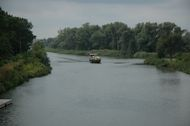Navigation: Home ›  The model ›  Special features ›  Spatial interpolation of meteorologic data ›  Thiessen Polygons

# Interpolation using Thiessen Polygons

The Thiessen polygons are a kind of special case of IDW interpolation. Only the nearest station is used for interpolating the data. Thus, the typical polygons are created when displaying the interpolation results as a map.

Interpolation method 4 is the pure Thiessen polygon method. Interpolation method 12, however, uses Thiessen polygons as basis to a somewhat more complex interpolation.

By adding additional parameters it is possible to combine the value of the nearest station with a specific lapse rate for up to two elevation ranges (given as parameters). If, for instance, the nearest station has an elevation of 1500 m a.s.l. and the actual cell has an elevation of 2400 m, then a lapse rate can be applied to regard the difference in altitude: a laspe rate of e.g. -0.007 would change the station value by an offset of (2400m -1500m)*(-0.007/m) = 900*(-0.007) = -6.3 (in this case it looks like a temperature gradient, but the method should rather be used for precipitation, since temperatures can be interpolated using the elevation dependent regression rather).

If the lapse rate changes significantly below or above a certain elevation, then even two different lapse rates (and the switching elevation) can be defined. Thus, it is possible to always get the data of the nearest station but to also consider the elevation dependency of an entity to some degree.

More details on Thiessen polygons method can be found in the WaSiM model documentation (chapter on interpolation).# WaSiM-News

05/15/2020

new release availabe:
WaSiM 10.06.00 -> More »